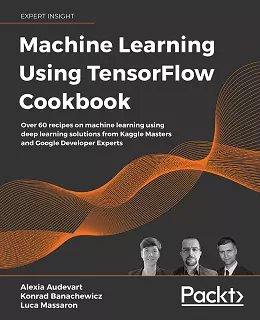# Machine Learning Using TensorFlow Cookbook### eBook Details:

• Paperback: 416 pages
• Publisher: WOW! eBook (February 8, 2021)
• Language: English
• ISBN-10: 1800208863
• ISBN-13: 978-1800208865

### eBook Description:

Machine Learning Using TensorFlow Cookbook: Master TensorFlow to create powerful machine learning algorithms, with valuable insights on Keras, Boosted Trees, Tabular Data, Transformers, Reinforcement Learning and more

The independent recipes in Machine Learning Using TensorFlow Cookbook will teach you how to perform complex data computations and gain valuable insights into your data. You will work through recipes on training models, model evaluation, sentiment analysis, regression analysis, artificial neural networks, and deep learning – each using Google’s machine learning library, TensorFlow.

This cookbook begins by introducing you to the fundamentals of the TensorFlow library, including variables, matrices, and various data sources. You’ll then take a deep dive into some real-world implementations of Keras and TensorFlow and learn how to use estimators to train linear models and boosted trees, both for classification and for regression to provide a baseline for tabular data problems.

As you progress, you’ll explore the practical applications of a variety of deep learning architectures, such as recurrent neural networks and Transformers, and see how they can be applied to computer vision and natural language processing (NLP) problems. Once you are familiar with the TensorFlow ecosystem, the final chapter will teach you how to take a project to production.

• Grasp linear regression techniques with TensorFlow 2
• Use Estimators to train linear models and boosted trees for classification or regression
• Execute neural networks and improve predictions on tabular data
• Master convolutional neural networks and recurrent neural networks through practical recipes
• Apply reinforcement learning algorithms using the TF-Agents framework
• Implement and fine-tune Transformer models for various NLP tasks
• Take TensorFlow 2 into production

By the end of this Machine Learning Using TensorFlow Cookbook book, you will be proficient in using TensorFlow 2. You’ll also understand deep learning from the fundamentals and be able to implement machine learning algorithms in real-world scenarios.

[ Read also: The Rise of Machine Learning on The Edge: AI Everywhere article on The Cloud Navigator ]